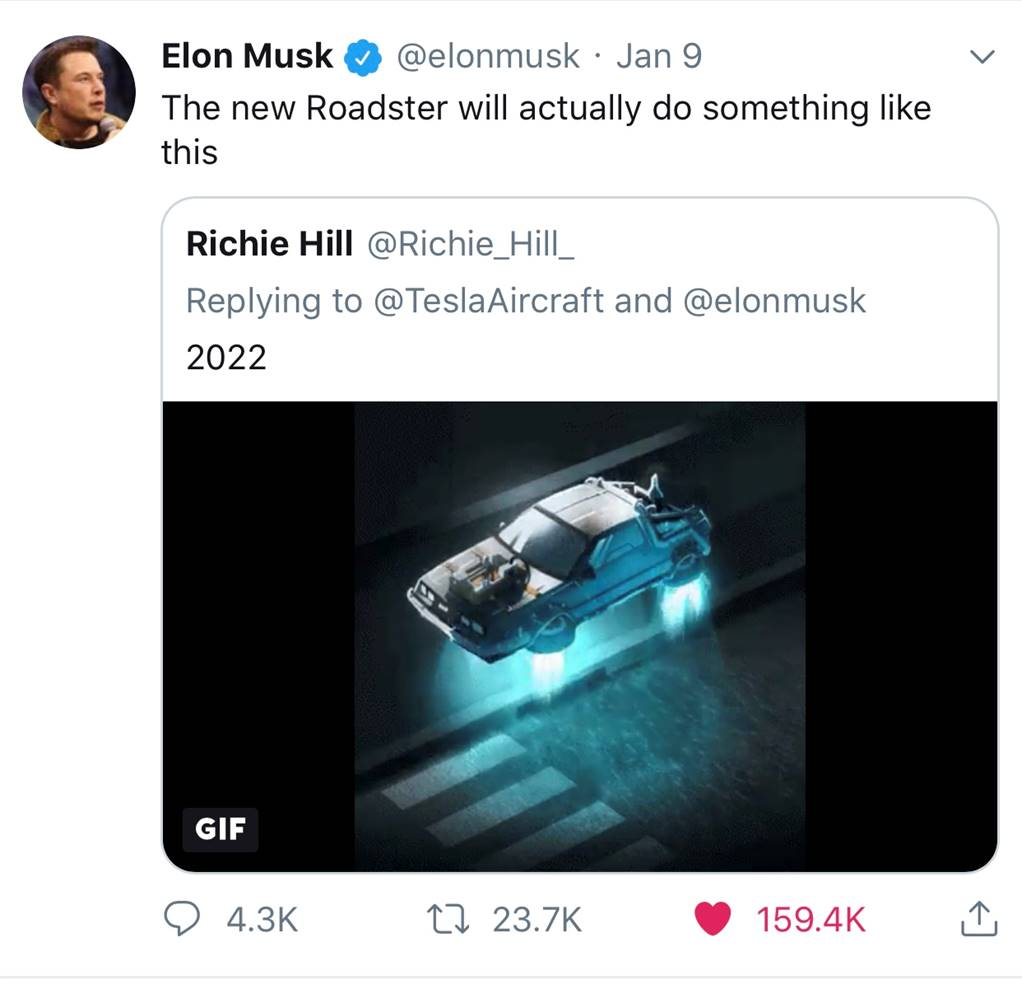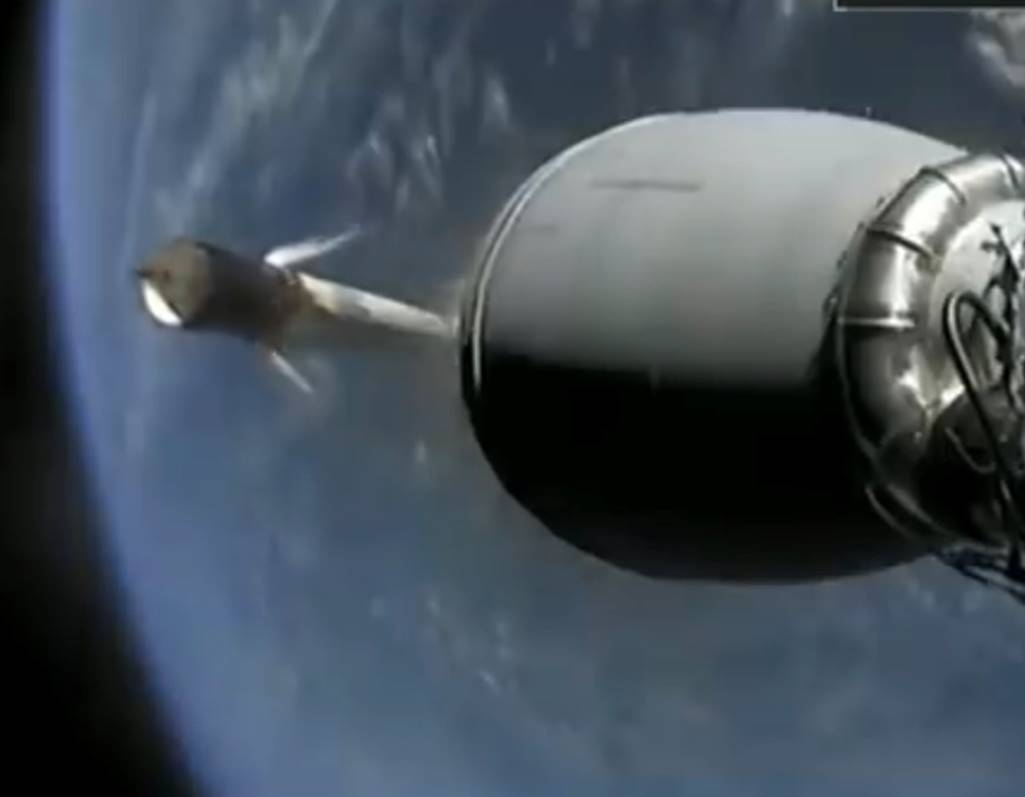Can SpaceX Really Make a Tesla Fly?Following the unveil of Tesla’s new roadster, Elon Musk tweeted it was just the base model, promising a ‘SpaceX’ version with thrusters to enhance performance that might even allow the car to fly. Many assumed he was joking, but in Feb 2021 he doubled down and added some technical details.

But is this even possible? Could SpaceX technology really make a Tesla Roadster fly (apart from on the end of Falcon Heavy)?

All the hot air from Journo’s with media studies degrees is not required: it’s just a basic Physics and rocketry problem. So I thought I’d whip out my digital envelope and scrawl some calculations on the back to see if I could get a more quantitive answer. Here goes …

First of all, what does Elon mean by ‘fly’. As I originally wrote in the first version of this article, by ‘fly’ he means rise a few metres and then hop short distances (maybe across a car park).

Musk has been quite specific about the technology and the performance envelope. We’re not talking fire-breathing rocket motors and orbital velocities here.

The SpaceX Tesla Roadster will employ cold gas thrusters blasting out compressed air stored in a composite overwrapped pressure vessel (or COPV). This is the same basic tech that SpaceX use to adjust the attitude of their returning Falcon 9 first stages (those puffs of gas you see here).Now, to find out if a Tesla can fly using those cold gas thrusters, we just need to figure out the amount of air required by the thrusters to keep the Roadster hovering and compare that with the capacity of a suitable COPV.

To get a Tesla to hover is going to require upward force (thrust): specifically, about the thrust required to just overcome the force of gravity (bit more going up, bit less going down). That force is given by the familiar equation:

F = Ma

In this case, to just support the car against gravity, that becomes:

F = MTesla x g

Where:

F is the force required in Newtons

MTesla is the Mass of the Tesla Roadster

g is the acceleration due to Earth’s gravity: 9.8 ms-2

Now to calculate the mass flow through those cold gas thrusters, we can use the specific impulse equation:

F = g x Isp x m

Where:

F is the force calculated above

Isp is the specific impulse

m is the mass flow rate through the thrusters

Substituting one equation into the other, we get

MTesla x g = g x Isp x m

Simplifying and rearranging, the mass flow, m, is then just:

m = MTesla / Isp

Wikipedia quotes the maximum specific impulse for a nitrogen thruster as 76s. Air is mostly nitrogen, so let’s just assume a round 70s. (Update Sept 2019: Elon just confirmed this figure for SpaceX nitrogen thrusters in a Starship presentation).

Thus we get:

m = 2000 Kg / 70s = 28 Kg/s

In other words, those SpaceX cold gas thrusters will need to emit very roughly 28 Kg of compressed air every second to keep our Roadster hovering.

Now let’s figure out how much air the COPV might hold. Looking at the dimensions of a Roadster and given the fact that the COPV will replace the rear seats, I reckon a cylindrical COPV with hemispherical ends of very roughly 2.0m x 1.0m should fit. That would conveniently have a volume of a bit more than one cubic metre.

To find out how much air the COPV could hold, we need to know its maximum pressure. My first version of this article estimated a max COPV pressure, based on old NASA tests, of around 2000 PSI. However, Elon has said recently (Feb 2021) ‘around 10,000 PSI’ – SpaceX has been doing lots of development work on COPVs.

From graphs I found online, air at a pressure of 10,000 PSI has a density of at least 500 Kg per cubic metre (depending on temperature).

If the COPV can hold about a 500 Kg of air then a Tesla Roadster might hover for about twenty seconds at 28 Kg/s mass flow through the thrusters, as calculated above.

In conclusion, I’d reckon that SpaceX CGT/COPV technology might allow 15-30 seconds of hover time for a Tesla Roadster, depending on the size and max pressure of the COPV, maybe a bit more if they really pushed things. With a few m/s of forward motion, a slow drift across a park would be easy.

SpaceX CGT tech really could make a Tesla fly, at least for short distances.

(Whether issues such as flying debris and noise would make this impractical, I can’t say).